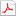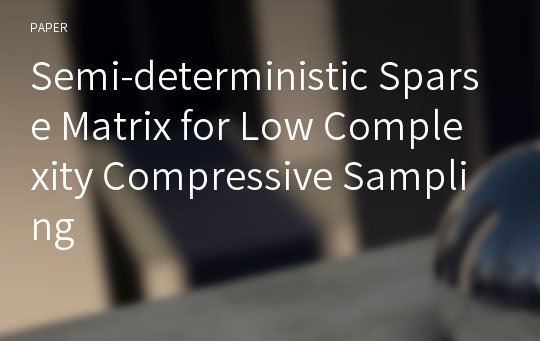# Semi-deterministic Sparse Matrix for Low Complexity Compressive Sampling

최초 등록일
2017.09.07
최종 저작일
2017.09
16페이지/어도비 PDF
가격
판매자한국학술정보(주)
다운로드
장바구니

* 본 문서는 배포용으로 복사 및 편집이 불가합니다.

## 서지정보

발행기관 : 한국인터넷정보학회 수록지정보 : KSII Transactions on Internet and Information Systems (TIIS) / 11권 / 5호
저자명 : ( Lei Quan ) , ( Song Xiao ) , ( Xiao Xue ) , ( Cunbo Lu )

## 영어 초록

The construction of completely random sensing matrices of Compressive Sensing requires a large number of random numbers while that of deterministic sensing operators often needs complex mathematical operations. Thus both of them have difficulty in acquiring large signals efficiently. This paper focuses on the enhancement of the practicability of the structurally random matrices and proposes a semi-deterministic sensing matrix called Partial Kronecker product of Identity and Hadamard (PKIH) matrix. The proposed matrix can be viewed as a sub matrix of a well-structured, sparse, and orthogonal matrix. Only the row index is selected at random and the positions of the entries of each row are determined by a deterministic sequence. Therefore, the PKIH significantly decreases the requirement of random numbers, which has a complex generating algorithm, in matrix construction and further reduces the complexity of sampling. Besides, in order to process large signals, the corresponding fast sampling algorithm is developed, which can be easily parallelized and realized in hardware. Simulation results illustrate that the proposed sensing matrix maintains almost the same performance but with at least 50% less random numbers comparing with the popular sampling matrices. Meanwhile, it saved roughly 15%-35% processing time in comparison to that of the SRM matrices.

없음

더보기 (5/10)

## 자료문의

제휴사는 별도로 자료문의를 받지 않고 있습니다.

## 판매자 정보한국학술정보(주)는 콘텐츠 제작에 도움이 되는 솔루션을 기반으로 풍부한 문화 콘텐츠를 생성하여 새로운 삶의 가치를 창조합니다.

본 학술논문은 한국학술정보(주)와 각 학회간에 저작권계약이 체결된 것으로 AgentSoft가 제공 하고 있습니다.
본 저작물을 불법적으로 이용시는 법적인 제재가 가해질 수 있습니다.최근 본 자료더보기